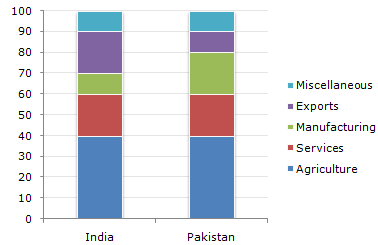# Data Interpretation - Bar Charts - Discussion

The following bar chart shows the composition of the GDP two countries (India and Pakistan).

Composition of GDP of Two Countries5.

If the total GDP is the same for both the countries, then what percentage is Pakistan's income through agriculture over India's income through Services ?

 [A]. 100 % [B]. 200 % [C]. 133.33 % [D]. None of these

Explanation:

Since the GDP is same, the answer will be got by (40 - 20)/20 = 100%.

 Rohit said: (Mar 5, 2011) I don't understand this. Can you please explain.

 Sukhjeet said: (May 11, 2011) Why we divide this number (40-20) by 20?

 Pranav said: (May 20, 2011) The correct answer is 200%. We should divide 40 by 20, and not (40-20) by 20. The question does not ask for the difference.

 Sundar said: (May 22, 2011) @All You should understand the line "Pakistan's income through agriculture OVER India's income". Just look the word 'OVER' ... (it means higher than) Let us compare Income ratio: India (by Services) = 20% Pakistan (by Agriculture) = 40% If Pakistan = 40, then India = 20 If Pakistan = 100 (40 x 2.5) then India = 50 (20 x 2.5) If Pakistan = 200 then India = 100 Reversed If INDIA = 100% then PAKISTAN = 200% Here Pakistan have 100% over income than India. One more example: Assume, India = 100 and Pakistan = 120, Then Pakistan have 20% higher/over income than India.

 Gopesh Debnath said: (Jun 4, 2011) Nice appreciation.

 Monika said: (Mar 3, 2012) Thanks Sundar.

 Ria Chopra said: (Apr 30, 2013) Hey they have not asked for percentage change for which we need to minus it. Its just asking the percentage of pakistan over India so we don't have to minus I guess. I'm confused can someone please give the right answer.

 Charan said: (Jun 12, 2013) Just look at the question it was asked to find what percentage is Pakistan's income through agriculture over India's income through Services ? By looking at the diagram we can infer, Pakistan's income through agriculture = 40. India's income through Services = 20. Just look at the word 'over' means 'additional to' or 'percentage increase' of pak's income in agriculture with that of india's income in services. Therefore we have to subtract india's income from pak's income. So it becomes: x= (40-20)/20*100=100%. That's it. Just try to understand guys :).

 Abrar said: (Oct 17, 2013) @Charan why did you divide by 20?

 Saji said: (Sep 2, 2015) Why we divide by 20 please answer?

 Ashwini said: (Aug 10, 2018) Percentage increase formula: (a-b)/b * 100%. (40-20)/20 * 100% = 100%.

 Sachin Mathivanan said: (Apr 19, 2019) I think the answer is 200%.

 Jalaj Kumar Bhardwaj said: (Aug 13, 2020) Well said, thanks @Charan.

 Sriya said: (Sep 1, 2020) @Sundar @Charan. X over Y means X divided by Y. So, the right answer must be 200.

 Pema said: (Oct 23, 2020) I think it is 200%.

 Tiger Ongchu said: (Aug 31, 2021) The right answer should be 200 not 100. The word 'over' means just to do with ratio or to place the given datas in numerator and denominator.

 Mohd Shariq said: (Sep 15, 2021) 200% should be the right answer.

 Mohd Shariq said: (Sep 15, 2021) 200% should be the right answer.